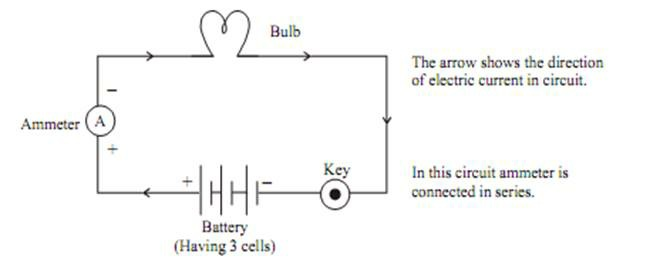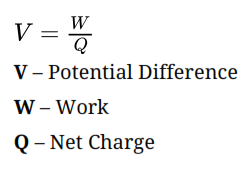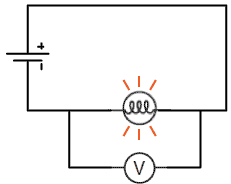# Ammeter

It is an instrument used to measure the electric current in a circuit. It is always connected in series in a circuit. It has low resistance(practically). Ideally ammeter’s resistance must be zero

## Electric Circuit for Ammeter

It is a closed path along which the electric current flows.• The electrons can only flow when there is the difference of electric potential.
• For example “water flowing through a tube”. It is only possible when there is high pressure at one side and low at another side, then it will move from high pressure to low pressure.
• In case of electric current, the flow of charge is made possible due to chemical action within a cell that generates the potential difference across the terminals of the cell.

# Electric potential Difference

It is defined as the work done in carrying a unit charge from one point to another in an electric circuit.SI unit of potential difference is Volts. Denoted by “V”. It is named after Alessandro Volta.

# One Volt

When 1 Joule of work is done in carrying one coulomb (1C)of charge from one point to another of a current carrying conductor then the potential difference is said to be IV.# Voltmeter

A voltmeter is an instrument, used to measure the potential difference and represented by the symbol V in an electric circuit. It is always connected in parallel across the points between which the potential difference is to be measured. It has high resistance.

# Circuit Diagram for VoltmeterA circuit diagram (electrical diagram, elementary diagram, electronic schematic) is a graphical representation of an electric circuit.